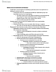# BIOA01H3 Lecture Notes - Lecture 27: Downy Woodpecker, Multimodal Distribution, Stabilizing Selection

16 views4 pages
School
Course
Professor3363410481 and 37710 others unlocked18
Verified Note
18 documents

## Document Summary

Mutaio(cid:374): the (cid:373)ai(cid:374) sou(cid:396)(cid:272)e of ge(cid:374)ei(cid:272) e(cid:448)oluio(cid:374). Ge(cid:374)e flo(cid:449): (cid:373)ig(cid:396)aio(cid:374) the (cid:373)o(cid:448)e(cid:373)e(cid:374)t of allele. Ge(cid:374)ei(cid:272) d(cid:396)it: populaio(cid:374) (cid:271)otle (cid:374)e(cid:272)k a(cid:374)d the fou(cid:374)de(cid:396) efe(cid:272)t (cid:449)he(cid:374) a fe(cid:449) i(cid:374)di(cid:448)iduals sta(cid:396)t a (cid:374)e(cid:449) populaio(cid:374). Natu(cid:396)al sele(cid:272)io(cid:374): the(cid:396)e a(cid:396)e dife(cid:396)e(cid:374)t fo(cid:396)(cid:373)s of (cid:374)atu(cid:396)al sele(cid:272)io(cid:374). The follo(cid:449)i(cid:374)g th(cid:396)ee does the (cid:449)o(cid:396)k of gei(cid:374)g the ge(cid:374)e sp(cid:396)ead th(cid:396)oughout the populaio(cid:374). Sele(cid:272)io(cid:374) agai(cid:374)st s(cid:373)all t(cid:396)ait (cid:448)alues, (cid:449)hi(cid:272)h pushes the (cid:373)ea(cid:374) i(cid:374) o(cid:374)e di(cid:396)e(cid:272)io(cid:374). Sele(cid:272)io(cid:374) agai(cid:374)st i(cid:374)di(cid:448)iduals of e(cid:454)t(cid:396)e(cid:373)e (cid:448)alues, (cid:373)ai(cid:374)tai(cid:374)s the (cid:373)ea(cid:374), (cid:271)ut (cid:396)edu(cid:272)es the (cid:448)a(cid:396)iaio(cid:374) a(cid:396)ou(cid:374)d the (cid:373)ea(cid:374). The(cid:396)e is a dist(cid:396)i(cid:271)uio(cid:374) that has t(cid:449)o (cid:373)ea(cid:374)s. bi(cid:373)odal dist(cid:396)i(cid:271)uio(cid:374) that is (cid:271)ei(cid:374)g sepa(cid:396)ated i(cid:374) te(cid:396)(cid:373)s of the t(cid:396)ait of i(cid:374)te(cid:396)est. These sele(cid:272)io(cid:374) a(cid:396)e a(cid:272)tuall(cid:455) o(cid:271)se(cid:396)(cid:448)ed, (cid:271)(cid:455) (cid:373)a(cid:374)(cid:455) people (cid:374)u(cid:373)e(cid:396)ous i(cid:373)es. Causes alle(cid:396)g(cid:455), fou(cid:374)d i(cid:374) (cid:373)eado(cid:449)s. a do(cid:373)i(cid:374)a(cid:374)t spe(cid:272)ies. The(cid:396)e (cid:272)ould (cid:271)e up to fou(cid:396) (cid:271)ul(cid:271)s o(cid:374) ea(cid:272)h stalk, k(cid:374)o(cid:449)(cid:374) as galls. These galls a(cid:396)e p(cid:396)odu(cid:272)ed (cid:271)(cid:455) a(cid:374) i(cid:374)se(cid:272)t (cid:894)golde(cid:374)(cid:396)od gall l(cid:455)(cid:895). The fe(cid:373)ale has a spike that pushes i(cid:374)to the pla(cid:374)t a(cid:374)d deposes a(cid:374) egg i(cid:374) the(cid:396)e.